# College Physics with Mastering Physics

Physics & Astronomy

## Quiz 8 : Rotational Motion and EquilibriumStudy FlashcardsLooking for Introductory Physics Homework Help?

## Quiz 8 :Rotational Motion and Equilibrium

Question TypeIn pure rotational motion of a rigid body, (a) all the particles of the body have the same angular velocity (b) all the particles of the body have the same tangential velocity, (c) acceleration is always zero, (d) there are always two simultaneous axes of rotation.
Free

A rigid body is an object or a system of particles in which the distance between any two particles is fixed.
Option (a) is correct answer because in pure rotational motion all of the particles of the object have the same instantaneous angular velocity.
Option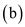is not correct because in pure rotational motion all the particles of a body have not the same tangential velocity because different particles are at different distances from the axis.
Option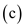is not correct because in pure rotational motion the acceleration is not always zero as the velocity of the particles changes continuously.
Option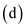is not correct because in pure rotational motion all particles in the body rotate about the same axis.

Tags
Choose question tagA ball with a radius of 15 cm rolls on a level surface, and the translational speed of the center of mass is 0.25 m/s. What is the angular speed about the center of mass if the ball rolls without slipping
Free
Essay

In pure rotational motion, all the particles in the object have same instantaneous angular velocity. The condition for rolling without slipping is,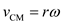Here, r is radius of object, and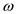is angular velocity.
Rearrange the equation for.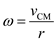The radius of ball is 15 cm. convert units of radius from cm to m.Substitute 0.25 m/s for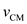, and 0.15 m for r in equationTherefore, the angular speed of ball is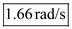.

Tags
Choose question tagA rolling object (a) has an axis of rotation through the axis of symmetry, (b) has a zero velocity at the point or line of contact, (c) will slip if s = r , (d) all of the preceding.
Free

Rolling is combination of translational and rotational motions. Adding the velocity vectors of these two motions shows that the point of contact is instantaneously at rest.
Option (a) is not correct because the axis of rotation changes with time in roiling motion.
Option (b) is correct because in rolling motion, the point of contact with surface is instantaneously at rest.
Option (c) is not correct if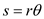, the body will role without slip.
Option (a) and option (c) are not correct, so potion (d) is not correct.

Tags
Choose question tagFor the tires on your rolling, but skidding car, (a) V CM = rw , (b) V CM rw (c) V CM rw (d) none of the preceding.
Tags
Choose question tagFor an object with only rotational motion, all particles of the object have the same (a) instantaneous velocity, (b) average velocity, (c) distance from the axis of rotation, (d) instantaneous angular velocity.
Tags
Choose question tagA bocce ball with a diameter of 6.00 cm rolls without slipping on a level lawn. It has an initial angular speed of 2.35 rad/s and comes to rest after 2.50 m. Assuming constant deceleration, determine (a) the magnitude of its angular deceleration and (b) the magnitude of the maximum tangential acceleration of the ball's surface (tell where that part is located).
Essay
Tags
Choose question tagIf the top of your automobile tire is moving with a speed of v , what is the reading of your speedometer
Essay
Tags
Choose question tagA bowling ball with a radius of 15.0 cm travels down the lane so that its center of mass is moving at 3.60 m/s. The bowler estimates that it makes about 7.50 complete revolutions in 2.00 seconds. Is it rolling without slipping Prove your answer, assuming that the bowler's quick observation limits answers to two significant figures.
Tags
Choose question tagIt is possible to have a net torque when (a) all forces act through the axis of rotation, (b)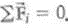, (c) an object is in rotational equilibrium, (d) an object remains in unstable equilibrium.
Tags
Choose question tagA rope goes over a circular pulley with a radius of 6.5 cm. If the pulley makes 4 revolutions without the rope slipping, what length of rope passes over the pulley
Essay
Tags
Choose question tag(a) When a disk rolls without slipping, should the product r be (1) greater than, (2) equal to, or (3) less than v CM (b) A disk with a radius of 0.15 m rotates through 270° as it travels 0.71 m. Does the disk roll without slipping Prove your answer.
Tags
Choose question tagA wheel rolls uniformly on level ground without slipping. A piece of mud on the wheel flies off when it is at the 9 o'clock position (rear of wheel). Describe the subsequent motion of the mud.
Essay
Tags
Choose question tagA wheel rolls 5 revolutions on a horizontal surface without slipping. If the center of the wheel moves 3.2 m, what is the radius of the wheel
Essay
Tags
Choose question tagFor a rolling cylinder, what would happen if the tangential speed v were less than rw Is it possible for v to be greater than rw Explain.
Essay
Tags
Choose question tagThe condition for rolling without slipping is (a) a c = rw 2 , (b) V CM = rw , (c) F = ma , (d) a c = v 2 / r.
Tags
Choose question tag"Popping a wheelie" is a motorcycle stunt in which the front end of the cycle rises up from the ground on a fast start, and can remain there for some distance. Explain the physics involved in this stunt.
Essay
Tags
Choose question tagSuppose someone in your physics class says that it is possible for a rigid body to have translational motion and rotational motion at the same time. Would you agree If so, give an example.
Essay
Tags
Choose question tagA small force and a large force produce torques. Can you tell which one will have the larger torque Explain.
Essay
Tags
Choose question tagIn cutting large trees, loggers first notch or make a V-cut on the side of the tree in the desired direction of fall and then cut from the other side. Why is this Is there any danger for the logger to be on the opposite side of the tree of the direction of fall
EssayExplain the balancing acts in Fig. Where are the centers of gravity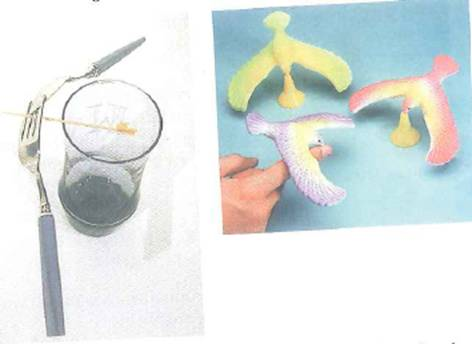FIGURE Balancing acts Left: A toothpick on the rim of the glass supports a fork and spoon. Right: Toy birds balance on their beaks. See Conceptual Question.